WEB前端之js只保留整数，向上取整，四舍五入，向下取整等函数

1.丢弃小数部分,保留整数部分
parseInt(5/2)

2.向上取整,有小数就整数部分加1

Math.ceil(5/2)

3,四舍五入.

Math.round(5/2)

4,向下取整

Math.floor(5/2)

Math 对象的方法

abs(x)    返回数的绝对值

acos(x)    返回数的反余弦值

asin(x)    返回数的反正弦值

atan(x)    以介于 -PI/2 与 PI/2 弧度之间的数值来返回 x 的反正切值

atan2(y,x)    返回从 x 轴到点 (x,y) 的角度（介于 -PI/2 与 PI/2 弧度之间）

ceil(x)    对一个数进行上舍入。

cos(x)    返回数的余弦

exp(x)    返回 e 的指数。

floor(x)    对一个数进行下舍入。

log(x)    返回数的自然对数（底为e）

max(x,y)    返回 x 和 y 中的最高值

min(x,y)    返回 x 和 y 中的最低值

pow(x,y)    返回 x 的 y 次幂

random()    返回 0 ~ 1 之间的随机数

round(x)    把一个数四舍五入为最接近的整数

sin(x)    返回数的正弦

sqrt(x)    返回数的平方根

tan(x)    返回一个角的正切

toSource()    代表对象的源代码

valueOf()    返回一个 Math 对象的原始值

js 四舍五入函数 toFixed（），里面的参数 就是保留小数的位数。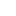喜欢 | 0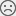不喜欢 | 0

• 33
文章
• 2818
人气
• 100%
受欢迎度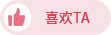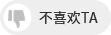•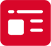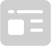索取资料
•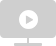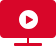答疑解惑
•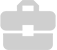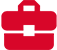技术交流
•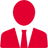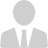职业测评
•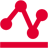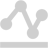面试技巧
•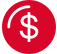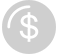高薪秘笈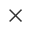Java全栈开发
WEB前端+H5沪公网安备 31011502005948号    ICP许可  沪B2-20190160